## The quotient as a modular inverse

We shall see now that the complexity result of Proposition 1 can be improved. To do so, we will show that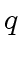can be computed from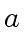and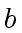by performing essentially one multiplication in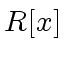. We start with the equation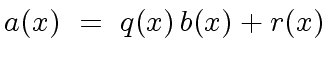(2)

where,,and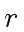are in the statement of Proposition 1. Replacing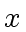by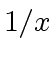and multiplying the equation by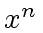leads to the new equation: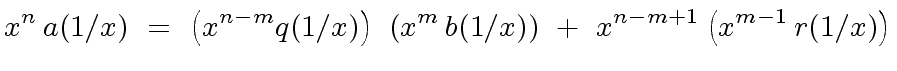(3)

In Equation (3) each of the rational fractions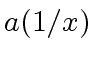,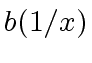,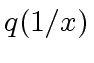and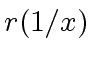is multiplied by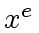such that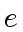is an upper bound for the degree of its denominator. So Equation (3) is in fact an equation in. Definition 1 and Proposition 2 highlight this fact. Then Proposition 3 and Theorem 1 suggest Algorithm 2 which provides an efficient way to compute the inverse of a univariate polynomial inmodulo a power of. Finally we obtain Algorithm 3 for fast division with remainder based on Equation (3).

Definition 1   For a univariate polynomial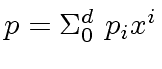inwith degree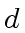and an integer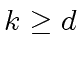, the reversal of order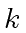of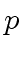is the polynomial denoted by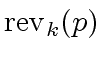and defined by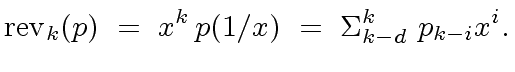(4)

When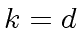the polynomialis simply denoted by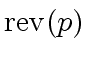. Hence we have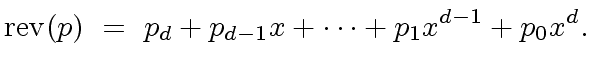(5)

Proposition 2   With,,andas abovce, we have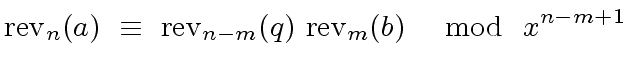(6)

Proof. Indeed with Definition 1 Equation (3) reads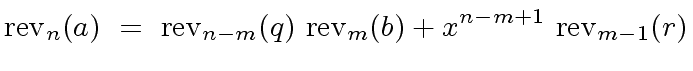(7)

leading to the desised result.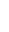Remark 2   If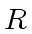is a field then we know that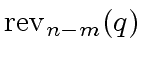is invertible modulo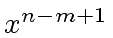. Indeed,has constant coefficient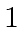and thus the gcd ofandis. The case whereis not a field leads also to a simple and surprising solution as we shall see in the next section.

Marc Moreno Maza
2008-01-07